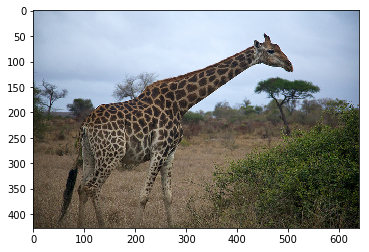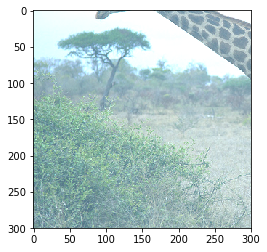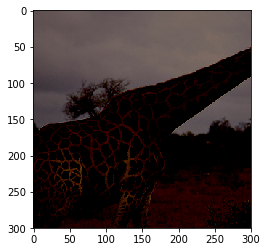# Methods of applying data augmentation (Gluon API)¶

Data Augmentation is a regularization technique that’s used to avoid overfitting when training Machine Learning models. Although the technique can be applied in a variety of domains, it’s very common in Computer Vision. Adjustments are made to the original images in the training dataset before being used in training. Some example adjustments include translating, cropping, scaling, rotating, changing brightness and contrast. We do this to reduce the dependence of the model on spurious characteristics; e.g. training data may only contain faces that fill 1/4 of the image, so the model trained without data augmentation might unhelpfully learn that faces can only be of this size.

In this tutorial we demonstrate a method of applying data augmentation with Gluon mxnet.gluon.data.Datasets, specifically the ImageFolderDataset.

%matplotlib inline
import mxnet as mx # used version '1.0.0' at time of writing
import numpy as np
from matplotlib.pyplot import imshow
import multiprocessing
import os

mx.random.seed(42) # set seed for repeatability


We define a utility function below, that will be used for visualising the augmentations in the tutorial.

def plot_mx_array(array):
"""
Array expected to be height x width x 3 (channels), and values are floats between 0 and 255.
"""
assert array.shape == 3, "RGB Channel should be last"
imshow((array.clip(0, 255)/255).asnumpy())

image_folder = os.path.join('data','images')

example_image = mx.image.imread(os.path.join(image_folder, "0.jpg")).astype("float32")
plot_mx_array(example_image)## Quick start with ImageFolderDataset¶

Using Gluon, it’s simple to add data augmentation to your training pipeline. When creating either ImageFolderDataset or ImageRecordDataset, you can pass a transform function that will be applied to each image in the dataset, every time it’s loaded from disk. Augmentations are intended to be random, so you’ll pass a slightly different version of the image to the network on each epoch.

We define aug_transform below to perform a selection of augmentation steps and pass it to our dataset. It’s worth noting that augmentations should only be applied to the training data (and not the test data), so you don’t want to pass this augmentation transform function to the testing dataset.

mxnet.image.CreateAugmenter is a useful function for creating a diverse set of augmentations at once. Despite the singular CreateAugmenter, this function actually returns a list of Augmenters. We can then loop through this list and apply each type of augmentation one after another. Although the parameters of CreateAugmenter are fixed, the random augmentations (such as rand_mirror and brightness) will be different each time aug_transform is called.

def aug_transform(data, label):
data = data.astype('float32')/255
augs = mx.image.CreateAugmenter(data_shape=(3, 300, 300),
rand_crop=0.5, rand_mirror=True, inter_method=10,
brightness=0.125, contrast=0.125, saturation=0.125,
pca_noise=0.02)
for aug in augs:
data = aug(data)
return data, label

training_dataset = mx.gluon.data.vision.ImageFolderDataset('data', transform=aug_transform)


We can quickly inspect the augmentations by indexing the dataset (which calls the __getitem__ method of the dataset). When this method is called (with an index) the correct image is read from disk, and the transform is applied. We can see the result of the augmentations when comparing the image below with the original image above.

sample = training_dataset
sample_data = sample
plot_mx_array(sample_data*255)In practice you should load images from a dataset with a mxnet.gluon.data.DataLoader to take advantage of automatic batching and shuffling. Under the hood the DataLoader calls __getitem__, but you shouldn’t need to call directly for anything other than debugging. Some practitioners pre-augment their datasets by applying a fixed number of augmentations to each image and saving the outputs to disk with the aim of increased throughput. With the num_workers parameter of DataLoader you can use all CPU cores to apply the augmentations, which often mitigates the need to perform pre-augmentation; reducing complexity and saving disk space.

batch_size = 1